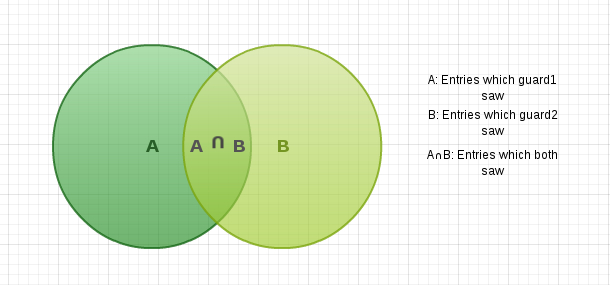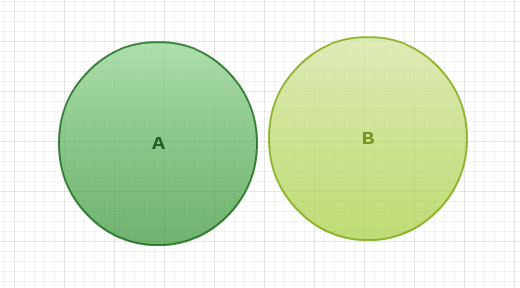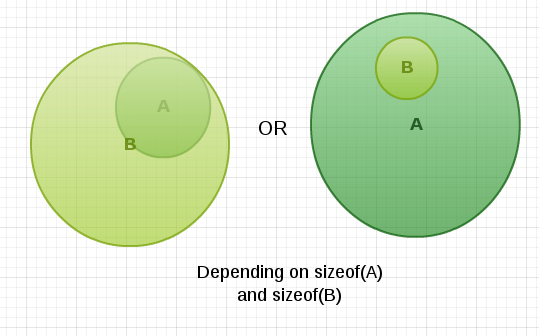# REMISS - Editorial

Tester: Tasnim Imran Sunny
Editorialist: Lalit Kundu

CAKEWALK

### PROBLEM:

Given the counts (A and B) of number of entries by two guards, what is the minimum and maximum number of entries that could have happened, given that atleast one of them is always awake.

### QUICK EXPLANATION:

Minimum occurs when they count as much as possible entries together. So the minimum would be the maximum(A,B).
Maximum occurs when each entry was counted only by one guard. So the maximum would be sum of counts of both i.e. A+B.

### EXPLANATION:A ∪ B is the total number of entries.
To maximise A ∪ B, make A ∩ B = 0, then A ∪ B = sizeof(A)+sizeof(B).To minimise A ∪ B, make any one set as a subset of other. Then the A ∪ B = maximum(sizeof(A),sizeof(B)).Pseudo code:

``````a,b=input()
print max(a,b),a+b
``````

### AUTHOR’S AND TESTER’S SOLUTIONS:

Author’s solution can be found here.
Tester’s solution can be found here.

5 Likes

@host why was my code incorrect?

@tanaymathur4
Hey you forgot to keep the endline character \n while printing output !
It should be
printf("%ld %ld\n",((a>b)?a:b),(a+b));

1 Like

is this a vaild mistake host?..come on dude…line changing is not a genuine mistake…your compiler should have accepted my code…

@tanaymathur4 Iam not the Adminstrator.I just looked at your code and told your mistake. All our submissions in codechef are judged by a machine . They are not done manually. So we have to be very careful. I had many such experiences in the past :). Good luck!@tanaymathur4, let’s have a look at output your code generates for
2
3 4
5 6

It would generate
4 76 11

Now how does a machine know you are trying to print “4 7\n6 11\n” even when to a human eye it looks completely wrong.

3 Likes

testcases = int(input())

# if testcases>=1 and testcases <= 100 :

for i in range(testcases):
if testcases >= 1 and testcases <= 100:
num1, num2 = input().split()
if int(num1) >= 0 and int(num1) <= 1000000 and int(num2) >= 0 and int(num2) < 1000000:
n = max(num2, num1)
maximum = int(num1) + int(num2)
# print(maximum)
print(n, maximum)

Can someone tell me please what is wrong with my code that the system says “wrong answer” ?

I was wrong and I was unkind to this problem, (the description though must change because is wrong)
My mistake was that my list, (arrays in python are called lists), was doing the sort() with my values as Strings so it gave me irrational results.
The same can happen with other python or ruby users if they are using array.sort() to do the sortingBest Regards
Robert

//